Documentation

## Reproduce Command Line or System Identification App Simulation Results in Simulink

Once you identify a model, you can simulate it at the command line, in the System Identification app, or in Simulink®. If you start with one simulation method, and migrate to a second method, you may find that the results do not precisely match. This mismatch does not mean that one of the simulations is implemented incorrectly. Each method uses a unique simulation algorithm, and yields results with small numeric differences.

Generally, command-line simulation functions such as `compare` and `sim`,as well as the System Identification app, use similar default settings and solvers. Simulink uses somewhat different default settings and a different solver. If you want to validate your Simulink implementation against command-line or app simulation results, you must ensure that your Simulink settings are consistent with your command-line or app simulation settings. The following paragraphs list these settings in order of decreasing significance.

### Initial Conditions

The most significant source of variation when trying to reproduce results is usually the initial conditions. The initial conditions that you specify for simulation in Simulink blocks must match the initial conditions used by `compare`, `sim`, or the System Identification app. You can determine the initial conditions returned in the earlier simulations. Or, if you are simulating against measurement data, you can estimate a new set of initial conditions that best match that data. Then, apply those conditions to any simulation methods that you use. For more information on estimating initial conditions, see Estimate Initial Conditions for Simulating Identified Models.

Once you have determined the initial conditions `x0` to use for a model `m` and a data set `z`, implement them in your simulation tool.

### Start Time of Input Data

In Simulink, the simulation default start time is zero, while in the app or command line the default start time is the sample time of the data.

Before exporting an `iddata` object for simulation in Simulink, set the object `Tstart` property to zero. Then export the object to Simulink using an Iddata Source block.

### Discretization of Continuous-Time Data

Simulink software assumes a first-order-hold interpolation on input data.

When using `compare`, `sim`, or the app, set the `InterSample` property of your `iddata` object to `'foh'`.

### Solvers for Continuous-Time Models

The `compare` command and the app simulate a continuous-time model by first discretizing the model using `c2d` and then propagating the difference equations. Simulink defaults to a variable-step true ODE solver. To better match the difference-equation propagation, set the solver in Simulink to a fixed-step solver such as `ode5`.

### Match Output of `sim` Command and Nonlinear ARX Model Block

Reproduce command-line `sim` results for an estimated nonlinear system in Simulink. When you have an estimated system model, you can simulate it either with the command-line `sim` command or with Simulink. The two outputs do not match precisely unless you base both simulations on the same initial conditions. You can achieve this match by estimating the initial conditions in your MATLAB model and applying both in the `sim` command and in your Simulink model.

Since you are directly comparing the `sim` and Simulink results, you also need to synchronize the timestamps of the first data points by setting the first time stamp in the data, `Tstart` to `0`. You set this time because, by default, command-line simulation assigns the sample time to the first timestamp, while Simulink assigns a timestamp of 0 seconds.

Load the estimation data you are using to identify a nonlinear ARX model. Create an `iddata` data object from the data. Specify the sample time as 0.2 seconds, and `Tstart` as 0 seconds.

```load twotankdata z = iddata(y,u,0.2,'Tstart',0); ```

Use the first 1000 data points to estimate a nonlinear ARX model `mw1` with orders [5 1 3] and wavelet network nonlinearity.

```z1 = z(1:1000); mw1 = nlarx(z1,[5 1 3],wavenet); ```

To initialize your simulated response consistently with the measured data, estimate an initial state vector `x0` from the estimation data `z1` using the `findstates` function. The third argument for `findstates` in this example, `Inf` , sets the prediction horizon to infinity in order to minimize the simulation error.

```x0 = findstates(mw1,z1,Inf); ```

Simulate the model `mw1` output using `x0` and the first 50 seconds (250 samples) of input data.

```opt = simOptions('InitialCondition',x0); data_sim = sim(mw1,z1(1:251),opt); ```

Now simulate the output of `mw1` in Simulink using a Nonlinear ARX Model block, and specify the same initial conditions in the block. The Simulink model `ex_idnlarx_block_match_sim` is preconfigured to specify the estimation data, nonlinear ARX model, initial conditions `x0`, and a simulation time of 50 seconds.

Open the Simulink model.

```model = fullfile(matlabroot,'examples','ident','ex_idnlarx_block_match_sim'); open_system(model); ```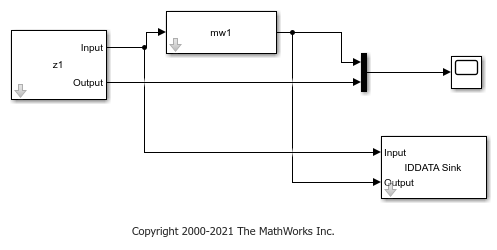Simulate the nonlinear ARX model output for 50 seconds.

```sim(model); ```

The IDDATA Sink block outputs the simulated output, `data`, to the MATLAB® workspace.

Plot and compare the simulated outputs you obtained using the `sim` command and Nonlinear ARX block.

```figure plot(data_sim.SamplingInstants,data_sim.OutputData,'b*',... data.SamplingInstants,data.OutputData,'-r') xlabel('Time(sec)'); ylabel('Simulated output'); legend('Output of sim command','Output of Nonlinear ARX block','location','southeast') title('Estimated Initial Conditions for Simulink') ```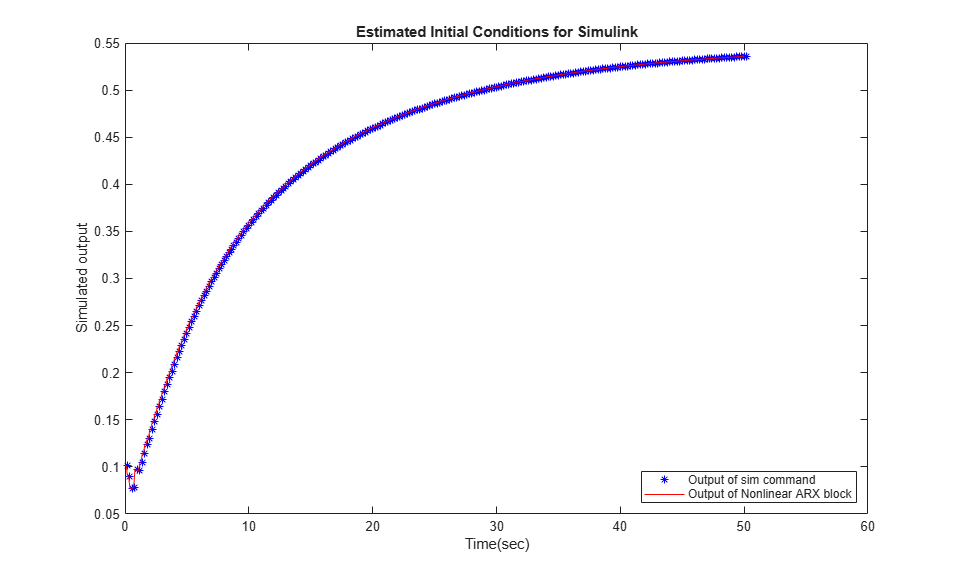The simulated outputs are the same because you specified the same initial condition when using `sim` and the Nonlinear ARX Model block.

If you want to see what the comparison would look like without using the same initial conditions, you can rerun the Simulink version with no initial conditions set.

Nullify initial-condition vector `x0`, and simulate for 50 seconds as before. This null setting of `x0` is equivalent to the Simulink initial conditions default.

```x0 = [0;0;0;0;0;0;0;0]; sim(model); ```

Plot the difference between the command-line and Simulink methods for this case.

```figure plot(data_sim.SamplingInstants,data_sim.OutputData,'b*',... data.SamplingInstants,data.OutputData,'-r') xlabel('Time(sec)'); ylabel('Simulated output'); legend('Output of sim command','Output of Nonlinear ARX block','location','southeast') title('Default Initial Conditions for Simulink') ```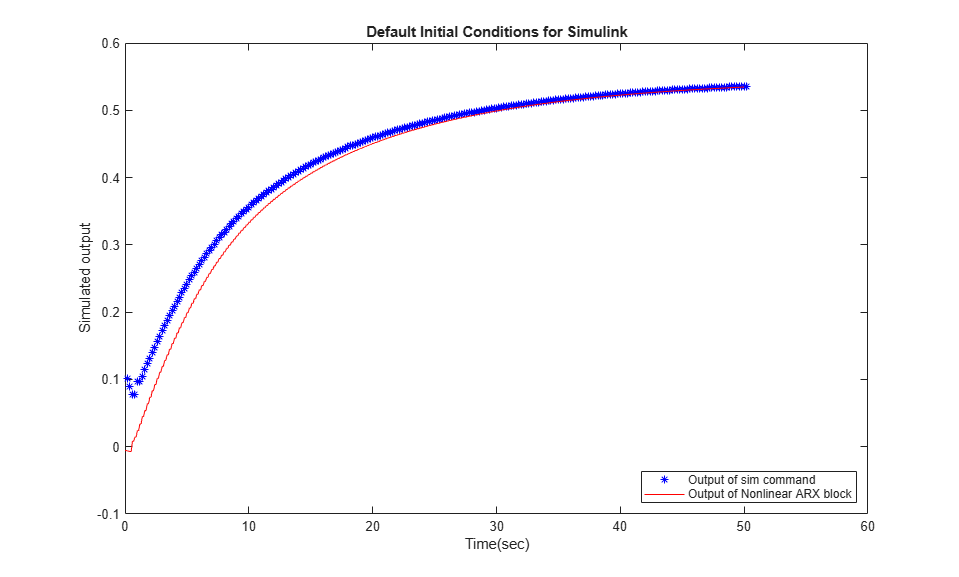The Simulink version starts at a different point than the `sim` version, but the two versions eventually converge. The sensitivity to initial conditions is important for verifying reproducibility of the model, but is usually a transient effect.

### Match Output of `compare` Command and Nonlinear ARX Model Block

In this example, you first use the `compare` command to match the simulated output of a nonlinear ARX model to measured data. You then reproduce the simulated output using a Nonlinear ARX Model block.

Load estimation data to estimate a nonlinear ARX model. Create an `iddata` object from the data. Specify the sample time as 0.2 seconds and the start time of the data as 0 seconds.

```load twotankdata z = iddata(y,u,0.2,'Tstart',0); ```

Use the first 1000 data points to estimate a nonlinear ARX model `mw1` with orders [5 1 3] and wavelet network nonlinearity.

```z1 = z(1:1000); mw1 = nlarx(z1,[5 1 3],wavenet); ```

Use the `compare` command to match the simulated response of `mw1` to the measured output data in `z1`.

```data_compare = compare(mw1,z1); ```

The `compare` function uses the first `nx` samples of the input-output data as past data, where `nx` is the largest regressor delay in the model. `compare` uses this past data to compute the initial states, and then simulates the model output for the remaining `nx+1:end` input data.

To reproduce this result in Simulink, compute the initial state values used by `compare`, and specify the values in the Nonlinear ARX Model block. First compute the largest regressor delay in the model. Use this delay to compute the past data for initial condition estimation.

```nx = max(getDelayInfo(mw1)); past_data = z1(1:nx); ```

Use `data2state` to get state values using the past data.

```x0 = data2state(mw1,z1(1:nx)); ```

Now simulate the output of `mw1` in Simulink using a Nonlinear ARX Model block, and specify `x0` as the initial conditions in the block. Use the remaining `nx+1:end` data for simulation.

```zz = z1(nx+1:end); zz.Tstart = 0; ```

The Simulink model `ex_idnlarx_block_match_compare` is preconfigured to specify the estimation data, nonlinear ARX model, and initial conditions.

Open the Simulink model.

```model = fullfile(matlabroot,'examples','ident','ex_idnlarx_block_match_compare'); open_system(model); ```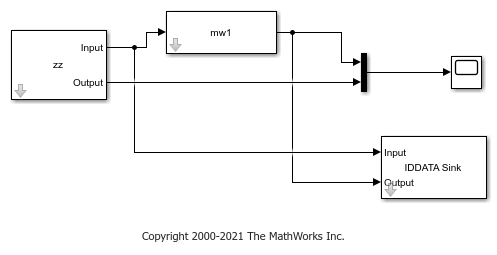Simulate the nonlinear ARX model output for 200 seconds.

```sim(model); ```

The IDDATA Sink block outputs the simulated output, `data`, to the MATLAB® workspace.

Compare the simulated outputs you obtained using the `compare` command and Nonlinear ARX block. To do so, plot the simulated outputs.

To compare the output of `compare` to the output of the Nonlinear ARX Model block, discard the first `nx` samples of `data_compare`.

```data_compare = data_compare(nx+1:end); data.Tstart=1; plot(data_compare,data,'r--') xlabel('Time(sec)'); legend('compare','Nonlinear ARX Model block') ```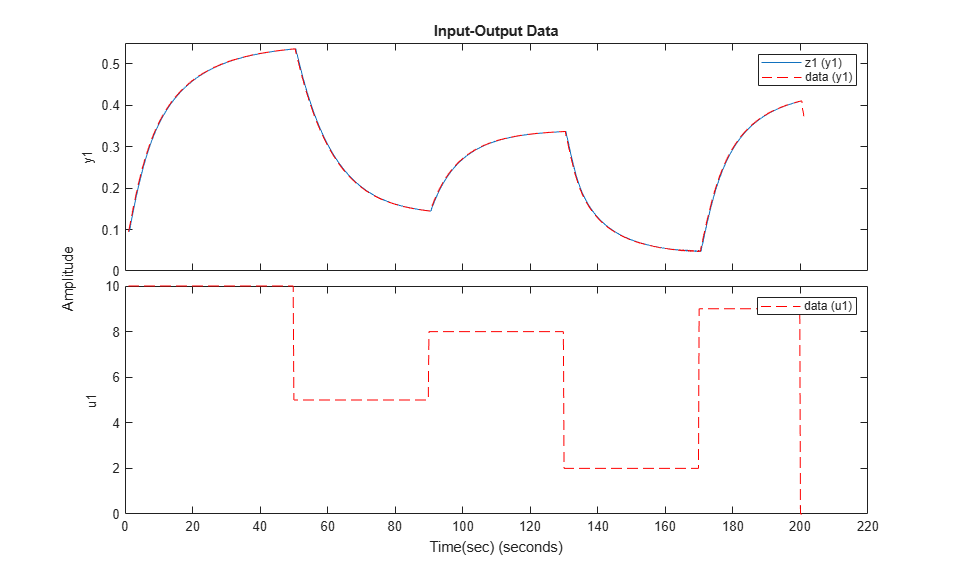The simulated outputs are the same because you specified the same initial condition when using `compare` and the Nonlinear ARX Model block.